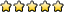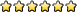## Astronomy C

Ashernoel
MemberPosts: 345
Joined: January 27th, 2017, 1:31 pm
State: IL
Location: MA
Has thanked: 0
Been thanked: 2 times

### Re: Astronomy C

embokim wrote:Suppose that now after a series of observations astronomers have deduced some properties of this pair of
stars. The parallax angle of this system is 0.0555…”. The mean separation of these two stars appears to
be 1.11”.

What is the semimajor axis of this binary star system, in AU?

How would you solve this question?
Thank You so much!
Ok so its a few steps but nothing crazy.

First you want to take the parallax angle and find the distance in parsecs to the system. d =1/theta.
Once you have the distance to the system, you can use the Small Angle formula to find the D, diameter in parsecs (or AU ish, there is a trick here to avoid conversions but lets keep it simple). 1.11" = 206265D/d

Now that you have the diameter of the system, the semi major axis is half of that and in AU, so use parsec to AU conversion and divide by 2. This problem makes a lot of assumptions about the observers being on earth and that the system's major axis is moving along a "transverse" line, but this method should work for the information that is given.
NT '19
Harvard '23

Unome
ModeratorPosts: 4269
Joined: January 26th, 2014, 12:48 pm
State: GA
Location: somewhere in the sciolyverse
Has thanked: 149 times
Been thanked: 51 times

### Re: Astronomy C

On the same test: can anyone explain how to do 2.a and 2.c?

(that was a great test btw, I ended up scoring ~55 or so).
Userpage
Chattahoochee High School Class of 2018
Georgia Tech Class of 2022

Opinions expressed on this site are not official; the only place for official rules changes and FAQs is soinc.org.

embokim
MemberPosts: 113
Joined: October 24th, 2013, 3:17 am
Division: C
State: NY
Location: between weird and genius
Has thanked: 0
Been thanked: 0

### Re: Astronomy C

Thank you so much! it helped me so much
But may I ask, what is the small angle formula?
Happy to be here

Ashernoel
MemberPosts: 345
Joined: January 27th, 2017, 1:31 pm
State: IL
Location: MA
Has thanked: 0
Been thanked: 2 times

### Re: Astronomy C

Unome wrote:On the same test: can anyone explain how to do 2.a and 2.c?

(that was a great test btw, I ended up scoring ~55 or so).
for 2a you look at the peak in B and see that it is slightly above 2446560 JD. Convert a value of around 2446562 JD to the Gregorian calendar and you should get around may 11th, 1986, which is probably pretty close.

As For 2c, either use the paper to reference the material, or get it wrong. The distance modulus used in the paper is used from Surface Brightness Fluctuations to the galaxy, which aren't given in the problem.

Hope this helps!
embokim wrote:Thank you so much! it helped me so much
AwesomeNT '19
Harvard '23

embokim
MemberPosts: 113
Joined: October 24th, 2013, 3:17 am
Division: C
State: NY
Location: between weird and genius
Has thanked: 0
Been thanked: 0

### Re: Astronomy C

For Newton's Law of Gravitation in what unit is R? (star separation distance)
Thanks
Happy to be here

Unome
ModeratorPosts: 4269
Joined: January 26th, 2014, 12:48 pm
State: GA
Location: somewhere in the sciolyverse
Has thanked: 149 times
Been thanked: 51 times

### Re: Astronomy C

embokim wrote:Thank you so much! it helped me so much
But may I ask, what is the small angle formula?
The small angle formula is an approximation of trigonometry. The basic concept is based upon something like this. (usually written as ) represents the linear diameter of the object being measured. This could be a single object, like the moon, or the separation between two stars in a binary system. (usually written as ) is the distance to the object, and is of course the angle. You can write as an equation representing the system. This works if and are measured in the same units, and if is measured in radians.

The small angle formula is an approximation for very large values of (and hence very small values of ) where the formula is approximately .

To get a more useful form of this equation, note that 1 radian = 206265 arcseconds, and 1 parsec = 206265 AU. This means that the function is also valid if is is AU, is in parsecs, and is in arcseconds. These are the units you're most likely to see in problems.
embokim wrote:For Newton's Law of Gravitation in what unit is R? (star separation distance)
Thanks
It can be in any unit, so long as G (the gravitational constant) is expressed in the same units; the units should cancel out to end up as units of force.
Ashernoel wrote:
Unome wrote:On the same test: can anyone explain how to do 2.a and 2.c?

(that was a great test btw, I ended up scoring ~55 or so).
for 2a you look at the peak in B and see that it is slightly above 2446560 JD. Convert a value of around 2446562 JD to the Gregorian calendar and you should get around may 11th, 1986, which is probably pretty close.
How do I convert? I can't find any reliable ways of doing it (and I'm not entirely sure how to derive the formula).
Userpage
Chattahoochee High School Class of 2018
Georgia Tech Class of 2022

Opinions expressed on this site are not official; the only place for official rules changes and FAQs is soinc.org.

CVMSAvalacheStudent
MemberPosts: 86
Joined: January 23rd, 2017, 8:49 pm
Division: C
State: CA
Location: Listening to the most boring lecture
Has thanked: 0
Been thanked: 0

### Re: Astronomy C

embokim wrote:Thank you so much! it helped me so much
But may I ask, what is the small angle formula?
I can't write it but you can search it up
"Great things in business are never done by one person. They're done by a team of people."-Steve Jobs

EastStroudsburg13Posts: 3166
Joined: January 17th, 2009, 7:32 am
State: MD
Pronouns: He/Him/His
Location: At work trying to be a real adult
Has thanked: 31 times
Been thanked: 135 times
Contact:

### Re: Astronomy C

embokim wrote:Thank you so much! it helped me so much
But may I ask, what is the small angle formula?
Short answer: if you have a small angle on a right triangle, the angle is approximately equal to the far side multiplied by the near side:So in the above, x = A/B. Note here that x is in radians, and A and B must be in the same units. Often, in astronomy, you'll be given arcseconds instead of radians, so you'll have to convert arcseconds to radians by multiplying by 206265 (units of arcsec/rad).

Now the long answer. Note: this may be a little confusing at first depending on your math background... I recommend drawing a little triangle and following along on your own if this is the case. You have been warned.The long answer involves explaining why the small angle formula works, which goes into a bit of trigonometry. In case you haven't seen trig yet, basically each trigonometric relation (sine, cosine, etc) is a ratio of two sides of a right triangle. So using the above angle x, the sine of x (sin x) is the length of the opposite side (A) divided by the length of the hypotenuse, the cosine of x (cos x) is the length of the adjacent side (B) divided by the length of the hypotenuse, and the tangent of x (tan x) is the length of A divided by the length of B.

Now, if x is really, really small, there are three approximations that arise: sin x = x, cos x = 1, and tan x = x (these are technically based on limits, which are part of calculus). Therefore, instead of saying tan x = A/B, you can say x = A/B, which is the small angle approximation that is used in the small angle formula.

The reason I went through this whole explanation is because the small angle approximation is used a lot in different areas of engineering, materials, and physics at the university level. You may not see it a ton in high school, depending on how advanced your school goes, but it's a good thing to be aware of by the time you encounter professors who are using it!

EDIT: Also see Unome's response
East Stroudsburg South Class of 2012, Alumnus of JT Lambert, Drexel University Class of 2017

Wiki
Wiki Pages that Need Work
FAQ and SciOly FAQ Wiki
BBCode Wiki

So long, and thanks for all the Future Dictator titles!

y_utsumi
MemberPosts: 6
Joined: August 2nd, 2016, 8:14 pm
Division: C
State: FL
Has thanked: 0
Been thanked: 0

### Re: Astronomy C

What is the difference among the 3 variants of Kepler's Law? They all produce different answers..

1. p^2 = a^3

2. p^2 = (4 pi^2 a^3) / (GM_total)

3. M_total = a^3 / p^2

EastStroudsburg13Posts: 3166
Joined: January 17th, 2009, 7:32 am
State: MD
Pronouns: He/Him/His
Location: At work trying to be a real adult
Has thanked: 31 times
Been thanked: 135 times
Contact:

### Re: Astronomy C

y_utsumi wrote:What is the difference among the 3 variants of Kepler's Law? They all produce different answers..

1. p^2 = a^3

2. p^2 = (4 pi^2 a^3) / (GM_total)

3. M_total = a^3 / p^2
3 only works if M_total is in solar masses, p is in earth years, and a is in AU.

If the system has a solar mass of 1 (i.e. our solar system), then 3 simplifies to 1.

2 is the true full form of the law, and the units can be anything as long as they cancel correctly. So for example, if your G is in Nm^2/kg^2, then your a has to be in meters and your p has to be in seconds.
East Stroudsburg South Class of 2012, Alumnus of JT Lambert, Drexel University Class of 2017